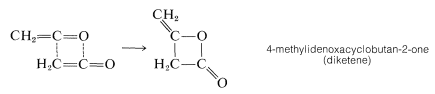# 17.7: Ketenes

$$\newcommand{\vecs}{\overset { \rightharpoonup} {\mathbf{#1}} }$$ $$\newcommand{\vecd}{\overset{-\!-\!\rightharpoonup}{\vphantom{a}\smash {#1}}}$$$$\newcommand{\id}{\mathrm{id}}$$ $$\newcommand{\Span}{\mathrm{span}}$$ $$\newcommand{\kernel}{\mathrm{null}\,}$$ $$\newcommand{\range}{\mathrm{range}\,}$$ $$\newcommand{\RealPart}{\mathrm{Re}}$$ $$\newcommand{\ImaginaryPart}{\mathrm{Im}}$$ $$\newcommand{\Argument}{\mathrm{Arg}}$$ $$\newcommand{\norm}{\| #1 \|}$$ $$\newcommand{\inner}{\langle #1, #2 \rangle}$$ $$\newcommand{\Span}{\mathrm{span}}$$ $$\newcommand{\id}{\mathrm{id}}$$ $$\newcommand{\Span}{\mathrm{span}}$$ $$\newcommand{\kernel}{\mathrm{null}\,}$$ $$\newcommand{\range}{\mathrm{range}\,}$$ $$\newcommand{\RealPart}{\mathrm{Re}}$$ $$\newcommand{\ImaginaryPart}{\mathrm{Im}}$$ $$\newcommand{\Argument}{\mathrm{Arg}}$$ $$\newcommand{\norm}{\| #1 \|}$$ $$\newcommand{\inner}{\langle #1, #2 \rangle}$$ $$\newcommand{\Span}{\mathrm{span}}$$$$\newcommand{\AA}{\unicode[.8,0]{x212B}}$$

## Preparation of Ketenes

Substances with cumulated carbonyl and carbon-carbon double bonds,, are called ketenes and, as may be expected, have interesting and unusual properties. Ketene itself, $$\ce{CH_2=C=O}$$, and its monosubstitution products, $$\ce{RCH=C=O}$$ ($$\ce{R} =$$ alkyl or aryl), are called aldoketenes, whereas disubstituted ketenes, $$\ce{R_2C=C=O}$$, are called ketoketenes.

There are relatively few general methods for preparing ketenes. The simplest procedure is to treat an $$\alpha$$-bromoacyl bromide with zinc, but the yields usually are not very good:Several special methods are available for the preparation of ketene itself. The most convenient laboratory preparation is to pass 2-propanone vapor over a coil of resistance wire heated electrically to a dull red heat; air is excluded to avoid simple combustion:The weakest bonds are the $$\ce{C-C}$$ bonds and, at $$750^\text{o}$$, fragmentation yields a methyl radical and an ethanoyl radical:Transfer of a hydrogen atom (i.e., disproportionation) gives methane and ketene. Industrially, ketene is best prepared by dehydration of ethanoic acid:## Reactions of Ketenes

Ketene has a boiling point of $$-56^\text{o}$$ and normally would be stored under pressure in steel cylinders. However, this is not possible because ketene is unstable with respect to formation of a dimer known as "diketene":The dimer also is a highly reactive substance with such unusual characteristics that its structure was not firmly established until 1956, almost 48 years after it first was prepared.

Ketenes in general are useful reagents for acylating alcohols, $$\ce{ROH}$$, and amines, $$\ce{RNH_2}$$, because the reactions involve additions; there are no by-products to be separated:Ketenes also can be used for the synthesis of cyclobutane derivatives through [2 + 2] cycloadditions with suitably active alkenes (Section 13-3D):Diketene is very useful in synthesis, particularly through its reactions with alcohols and amines to give derivatives of 3-oxobutanoic acid: